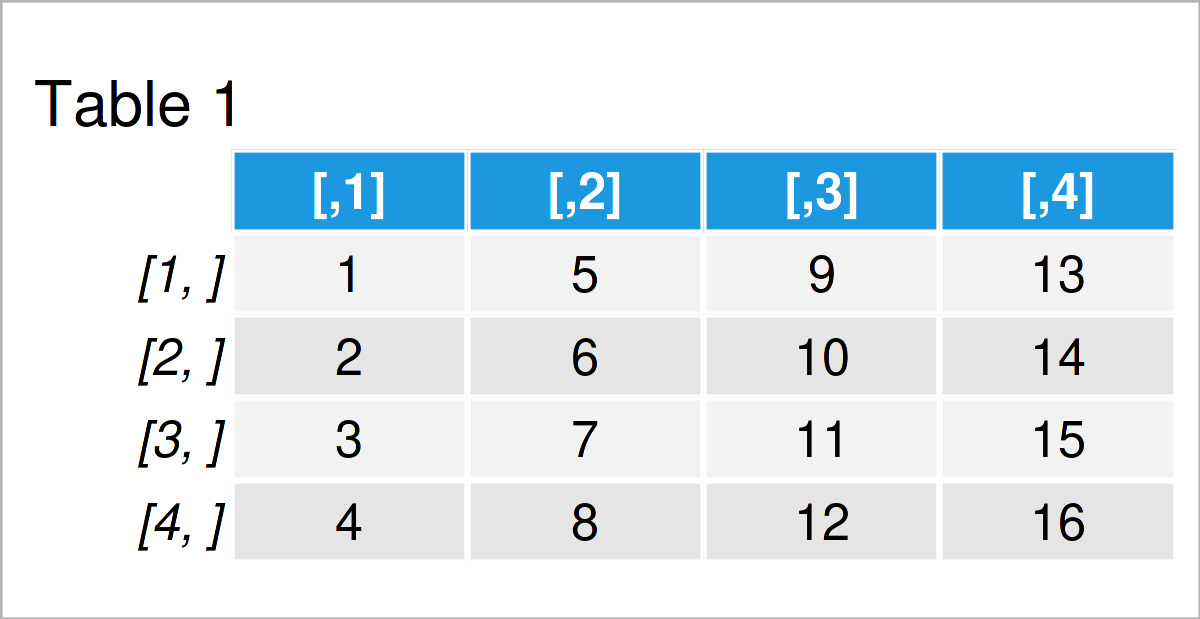# Modify Diagonal, Lower & Upper Triangular Part of Matrix in R (3 Examples)

This post shows how to change the diagonal, lower, and upper triangular part of a matrix in the R programming language.

The post will contain this information:

Let’s do this.

## Creating Exemplifying Data

I use the following data as basement for this R tutorial:

```my_mat <- matrix(1:16, ncol = 4)                  # Create example matrix
my_mat                                            # Print example matrix```Table 1 shows the structure of our example data – It contains four lines and four numerical columns.

## Example 1: Change Lower Triangular Part of Matrix Using lower.tri() Function

In this example, I’ll demonstrate how to apply the lower.tri function to modify the lower triangular values in a matrix object.

Let’s assume that we want to replace all values in the lower part of our matrix to zero.

Then, we can apply the lower.tri function as shown below:

```my_mat_new1 <- my_mat                             # Duplicate matrix
my_mat_new1[lower.tri(my_mat_new1)] <- 0          # Change lower triangular part
my_mat_new1                                       # Print updated matrix```Table 2 reveals the output of the previous R programming code: A new matrix where the entire lower part has been set to zero.

## Example 2: Change Upper Triangular Part of Matrix Using upper.tri() Function

Example 2 shows how to apply the upper.tri function to replace the values in the upper triangular matrix.

In this example, we’ll substitute the values in the input matrix by new values:

```my_mat_new2 <- my_mat                             # Duplicate matrix
my_mat_new2[upper.tri(my_mat_new2)] <- 101:106    # Change upper triangular part
my_mat_new2                                       # Print updated matrix```Table 3 shows the output of the previous R code: As you can see, we have inserted new values at each position of the upper part in our matrix.

## Example 3: Change Diagonal of Matrix Using diag() Function

In Example 3, I’ll explain how to adjust the diagonal values in a matrix using the diag function.

More precisely, the following R code inserts NA values for the entire diagonal:

```my_mat_new3 <- my_mat                             # Duplicate matrix
my_mat_new3[diag(my_mat_new3)] <- NA              # Change diagonal of matrix
my_mat_new3                                       # Print updated matrix```In Table 4 you can see that we have created another matrix containing missing data in the diagonal by running the previous R programming code.

## Video & Further Resources

As you have seen in this tutorial, the lower.tri, upper.tri, and diag functions can be used to modify the entries in a matrix object.

Would you like to learn more about the modification of the diagonal, lower, and upper triangular part of a matrix? Then you could watch the following video which I have published on my YouTube channel. In the video, I’m explaining the R code of this article in a live session.

Please accept YouTube cookies to play this video. By accepting you will be accessing content from YouTube, a service provided by an external third party.If you accept this notice, your choice will be saved and the page will refresh.

In addition, you may want to read the related R articles on my homepage. I have published several tutorials that are related to the modification of the diagonal, lower, and upper triangular part of a matrix already.

In this post you have learned how to replace the values in the diagonal, lower, and upper triangular part of a matrix in the R programming language. Let me know in the comments, in case you have further comments and/or questions. Furthermore, please subscribe to my email newsletter to receive regular updates on the newest articles.

Subscribe to the Statistics Globe Newsletter